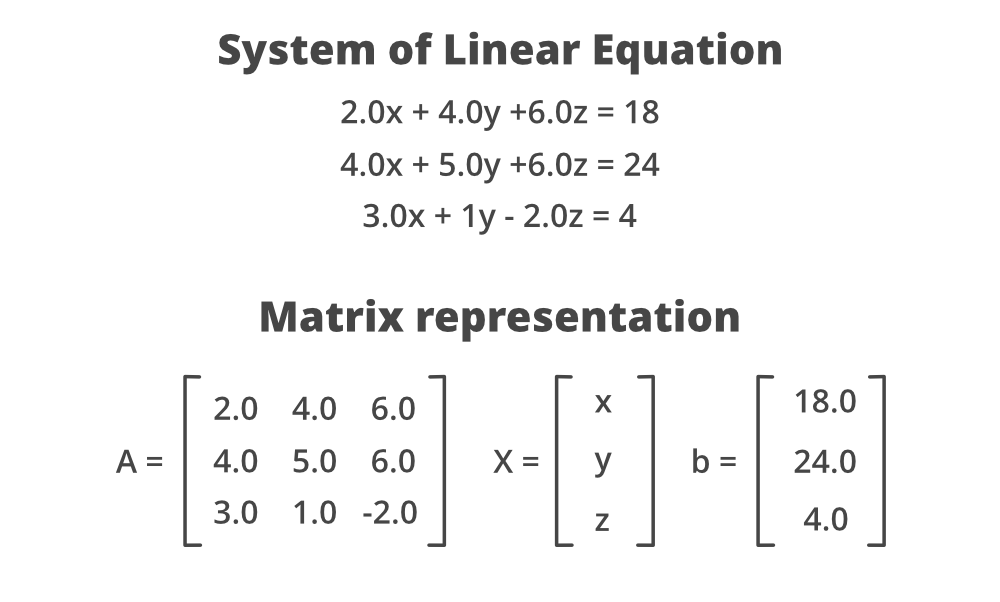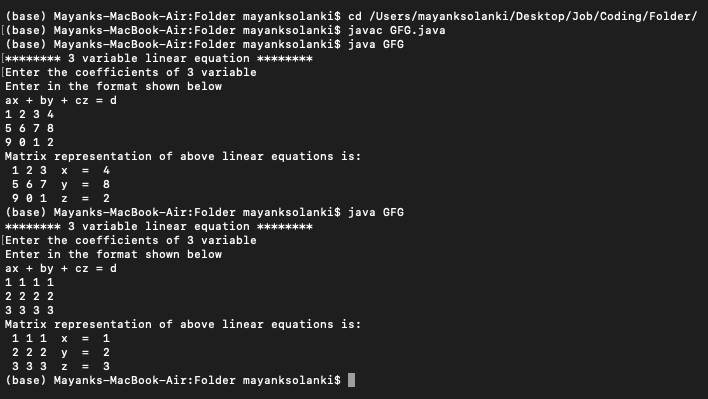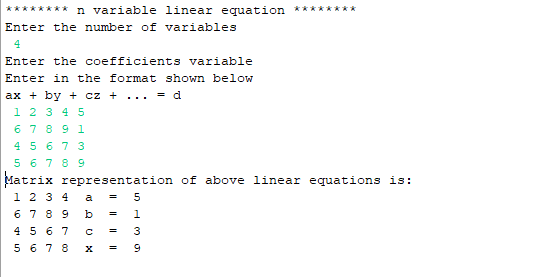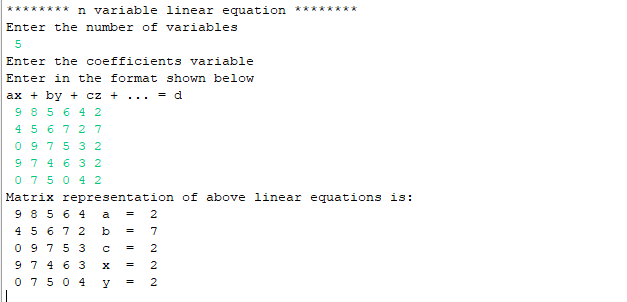Java Program to Represent Linear Equations in Matrix Form

• Last Updated : 30 Sep, 2021

Let’s look at the System of Linear Equation with the help of an example:The input of coefficients and variables is taken into play for consideration.

1. The scanner package should be imported into the program in order to use the object of the Scanner class to take the input from the user.
2. The array will be initialized to store the variables of the equations.
3. The coefficients of the variables will be taken from the user with the help of the object of the Scanner class.
4. The equations will then converted into the form of a matrix with the help of a loop.

Two examples are laid off:

1. 3 variable linear equations in matrix form.
2. N variable linear equations in matrix form.

Illustration: Considering the most used practical linear equation used in mathematics, that is 3 variable linear equations.

Input: ax + by + cz = d

Output - 1 2 3 x = 10
5 1 3 y = 12
7 4 2 z = 20

Example 1: Java Program for 3 variable linear equations in matrix form.

Java

 // Java Program to Represent Linear Equations in Matrix Form // Importing Scanner class// to take input from userimport java.util.Scanner; public class GFG {       // Mai driver method    public static void main(String args[])    {        // Display message for better readability        System.out.println(            "******** 3 variable linear equation ********");         // 3 variables of the linear equation        char[] variable = { 'x', 'y', 'z' };         // Creating Scanner class object        Scanner sc = new Scanner(System.in);         // Display message for asking user to enter input        System.out.println(            "Enter the coefficients of 3 variable");        System.out.println(            "Enter in the format shown below");        System.out.println("ax + by + cz = d");         // For 3*3 matrix or in other words        // Dealing with linear equations of 3 coefficients         // Input of coefficients from user        int[][] matrix = new int;        int[][] constt = new int;         // Outer loop for iterating rows        for (int i = 0; i < 3; i++) {            // Inner loop for iterating columns            for (int j = 0; j < 3; j++) {                 // Reading values from usr and                // entering in the matrix form                matrix[i][j] = sc.nextInt();            }             // One row input is over by now            constt[i] = sc.nextInt();        }         // The linear equations in the form of matrix         // Display message        System.out.println(            "Matrix representation of above linear equations is: ");         // Outer loop for iterating rows        for (int i = 0; i < 3; i++) {             // Inner loop for iterating columns            for (int j = 0; j < 3; j++) {                 // Printing matrix corresponding                // linear equation                System.out.print(" " + matrix[i][j]);            }             System.out.print("  " + variable[i]);            System.out.print("  =  " + constt[i]);            System.out.println();        }         // Close the stream and release the resources        sc.close();    }}

Output:Now, getting it generic for any value of N: “n-variable linear equation”

Illustration:

Input: ax + by + cz + ... = d

Output: 1 2 3 x = 10
5 1 3 y = 12
7 4 2 z = 20
...
...

Example 2: Java Program for N variable linear equations in matrix form.

Java

 import java.util.Scanner; public class Linear_Equations_n {    public static void main(String args[])    {        System.out.println(            "******** n variable linear equation ********");        // Initializing the variables        char[] variable            = { 'a', 'b', 'c', 'x', 'y', 'z', 'w' };        System.out.println("Enter the number of variables");        Scanner sc = new Scanner(System.in);        int num = sc.nextInt();        System.out.println(            "Enter the coefficients variable");        System.out.println(            "Enter in the format shown below");        System.out.println("ax + by + cz + ... = d");               // Input of coefficients from user        int[][] matrix = new int[num][num];        int[][] constt = new int[num];        for (int i = 0; i < num; i++) {            for (int j = 0; j < num; j++) {                matrix[i][j] = sc.nextInt();            }            constt[i] = sc.nextInt();        }        // Representation of linear equations in form of        // matrix        System.out.println(            "Matrix representation of above linear equations is: ");        for (int i = 0; i < num; i++) {            for (int j = 0; j < num; j++) {                System.out.print(" " + matrix[i][j]);            }            System.out.print("  " + variable[i]);            System.out.print("  =  " + constt[i]);            System.out.println();        }        sc.close();    }}

Output –4 – variable linear equations5 – variable linear equations

My Personal Notes arrow_drop_up